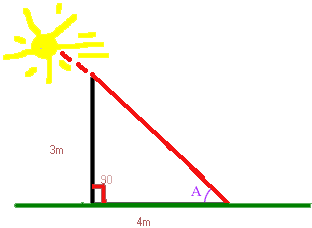--- Jeffrey My Problem is I don't understand how to do this problem. Here it is. At a Certain time, a vertical pole 3m tall cast a 4m shadow. What is the angle of elevation of the sun? Hi Jeffrey, The angle of elevation of the sun is the angle that I have labeled A in your diagram.If you know some trigonometry you will see that the tangent of the angle A is 3/4. You can then find the measure of the angle A by using the inverse tangent function on a calculator. Cheers, Harley Go to Math Central